### Home > CC1 > Chapter 7 > Lesson 7.3.2 > Problem7-99

7-99.
1. Simplify each of the following expressions. Homework Help ✎

1.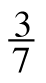÷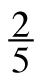2.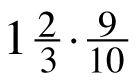3. 45 − 2(6 + 4 · 3)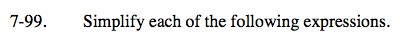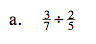Remember: one way to divide fractions is to find the reciprocal of the divisor (the second fraction) and then use the inverse operation, multiplication.
Multiply fractions by multiplying the numerators and denominators together, as shown below.

$\frac{a}{b}\cdot\frac{c}{d}=\frac{a\cdot\ c}{b\cdot\ d}$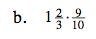Convert the mixed number to a fraction greater than one before multiplying.

For help finding the product of two fractions, see part (a).

$\frac{3}{2}=1\frac{1}{2}$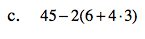Remember to follow the Order of Operations!
Try circling the terms then simplifying.

9